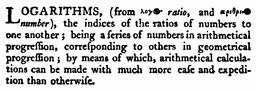# Logarithms: what are they?

A not so helpful definition of logarithms from 1797 Britannica:By Colin Macfarquhar (editor) [Public domain], via Wikimedia Commons

Logarithms are another way to consider unknown exponents. They ask: To what exponent do we raise this base to get another number?

## Here are a few examples and explanations:

Log2(8) = x

We interpret this as asking: “What number do we raise 2 to, to get 8”. So, let’s convert this into exponential form: 2x=8. We know that 2*2*2=8 so 23=8. Thus, x=3.

If a problem simply has log without a base, it is assumed that the base is 10. Thus: log 100=x is the same as log10100=x. In exponential form: 10x=100, thus x=2.

If we see a problem including ln (1)=x, it means the same thing as loge1=x. So, converting to exponential form, ex=1.  “e” is just a number, 2.71…. that goes on forever and is on most calculators. We know that any number raised to the 0 power is 1, so e0=1, or x=0.

Ln(x)=loge(x)By Krishnavedala (Own work) [CC BY-SA 3.0 (http://creativecommons.org/licenses/by-sa/3.0) or GFDL (http://www.gnu.org/copyleft/fdl.html)], via Wikimedia Commons